# ISEE Middle Level Math : How to find the part from the whole

## Example Questions

← Previous 1 3 4 5 6 7

Alice received $2.40 for her weekly allowance. If her favorite snacks cost 60¢ each, how many snacks can Alice buy this week? Possible Answers:Correct answer:Explanation: To solve: Divide the total amount of Alice's allowance ($2.40) by the price for each snack ($.60)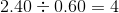Alice would be able to buy 4 snacks. ### Example Question #2 : How To Find The Part From The Whole Steve ate 20% of his pie on Monday. On Tuesday Steve ate 50% of the left-over pie. What percent of the pie is left? Possible Answers: 60% 30% 10% 20% 40% Correct answer: 40% Explanation: On Tuesday, Steve ate 50% (half) of the 80%, which is 40%. Steve has eaten 20% on Monday and 40% on Tuesday, so there is 40% left over. ### Example Question #3 : How To Find The Part From The Whole As a swim team member, Nicolas needs to train 90 hours a month. How many hours should Nicolas train each day of the 30-day month, in order to reach his goal? Possible Answers: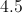Correct answer:Explanation: Divide 90 hours by 30 days in the month: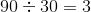Answer: Nicolas needs to train 3 hours a day. ### Example Question #4 : How To Find The Part From The Whole Brian found a motorcycle on sale for% off. If the original price of the motorcycle is$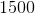, how much will Brian save?

$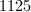$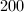$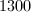$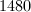$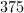Correct answer:$Explanation:

In order to find how much money Brian saved, we my first calculae% of $. We do this by multiplying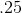by: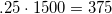Since we are looking for how much Brian saved and not how much he spent, our final answer is$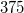.

### Example Question #5 : How To Find The Part From The Whole

An eight slice pizza pie must be shared evenly among four friends. How many slices will each person get?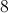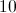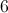Explanation:

Divide: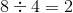Answer: Each person must get 2 slices.

### Example Question #6 : How To Find The Part From The Whole

A teacher has sixty stickers to give out to her 15 students. How many stickers will each student receive?Explanation:

Divide: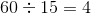### Example Question #7 : How To Find The Part From The Whole

Sixteen balloons are to be shared evenly among four children. How many balloons will each child get?Explanation:

Divide: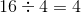balloons

### Example Question #8 : How To Find The Part From The Whole

Seventy people need to make five equal lines. How many people will be on each line?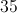Explanation:

Divide: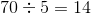people per line

### Example Question #9 : How To Find The Part From The Whole

Angelina goes to the grocery store. She only has $13.50 to spend. How many gallons of milk can she buy if each costs$4.50?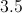Explanation:

Divide: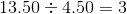Diana sold seven of her collectable dolls and made $350.00. How much was each doll sold for? Possible Answers: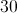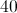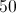Correct answer:Explanation: Divide: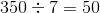Answer: Each doll was sold for$50.00.